# Quantity based Quiz for IBPS , SBI , RBI: Quiz – 3

## Quantity based Quiz for IBPS , SBI , RBI: Quiz – 3

Quantity Based Questions are one of the topics of Quantitative Aptitude Section that is asked in government exams. To answer the Quantity Based Questions, you must first understand all of the concepts. In this article, we have come up with the Quantity Based Quiz to enhance your preparation. Each question in this Quantity Based Quiz has a full explanation. This Quantity Based Quiz is based on the current or updated exam pattern. This Quantity Based Quiz is completely free of cost. This Quantity Based Quiz contains questions that range in difficulty from easy to challenging. Candidates must solve this Quantity Based Quiz in order to boost their preparation for upcoming exams.

Directions (1-10): In the given questions, two quantities are given, one as Quantity I and another as Quantity II. You have to determine relationship between two quantities and choose the appropriate option.
1. Quantity I = ‘x’ — A, B and C can complete a work in 15, 9 and 45 days respectively. All three starts working together. B worked for starting 2 days and left the work. After 3 more days C left the work. Remaining work is completed by A alone in ‘x’ days.
Quantity II = ‘y’ — y = 6
(a) Quantity I > Quantity II
(b) Quantity I < Quantity II
(c) Quantity I ≥ Quantity II
(d) Quantity I ≤ Quantity II
(e) Quantity I = Quantity II or No relation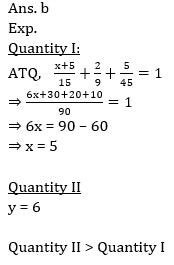2. Quantity I = ‘x’ — Ratio of speed of train X and train Y is 2 : 3. Length of train X and train Y is 200 m and 400 m respectively. ‘x’ is the speed of train X (in m/sec) while train X can cross trains Y coming from opposite direction in 6 secs.
Quantity II = ‘y’ — Train ‘P’ having speed y (in m/sec) can cross a pole in 2 seconds while it can cross two poles 120m apart from each other in 6 seconds.
(a) Quantity I > Quantity II
(b) Quantity I < Quantity II
(c) Quantity I ≥ Quantity II
(d) Quantity I ≤ Quantity II
(e) Quantity I = Quantity II or No relation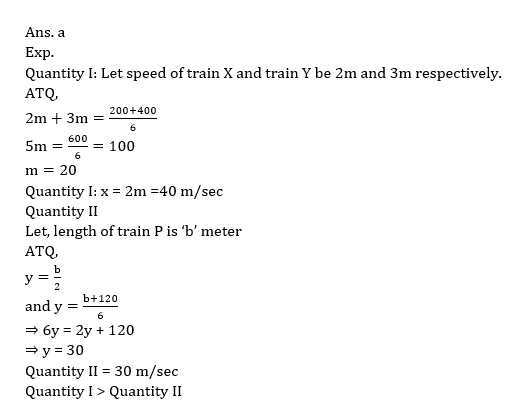3. Quantity I: = ‘x’ — A container contains 3 red, 4 green, 3 blue balls. ‘x’ is the probability of choosing two balls of same color from the container.
Quantity II = ‘y’ — y = 6/25
(a) Quantity I > Quantity II
(b) Quantity I < Quantity II
(c) Quantity I ≥ Quantity II
(d) Quantity I ≤ Quantity II
(e) Quantity I = Quantity II or No relation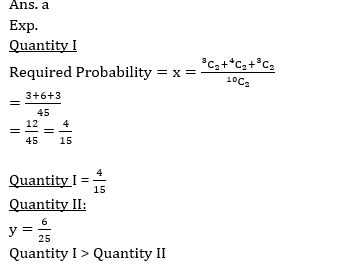4. Quantity I = ‘x’ — Retailer marked an article 25% above its cost price and gave discount of 12.5% at the time of sale and sell at Rs. 350. ‘x’ is the difference between marked price and cost price (in Rs.)
Quantity II = ‘y’ — Cost price of pen and pencil is in the ratio 2 : 3. Retailer earn overall 40% profit on selling one pen and one pencil together. But it was found that Pen is sold at cost price of pencil while pencil is sold at a profit Rs.40. ‘y’ is the cost price of pen (in Rs.)
(a) Quantity I > Quantity II
(b) Quantity I < Quantity II
(c) Quantity I ≥ Quantity II
(d) Quantity I ≤ Quantity II
(e) Quantity I = Quantity II or No relation5. Quantity I = ‘x’ — ‘x’ is the average of first six consecutive positive integral multiple of 4.
Quantity II = ‘y’ — 8 men can complete a work in 30 days while ‘y’ men can complete same work in 20 days.
(a) Quantity I > Quantity II
(b) Quantity I < Quantity II
(c) Quantity I ≥ Quantity II
(d) Quantity I ≤ Quantity II
(e) Quantity I = Quantity II or No relation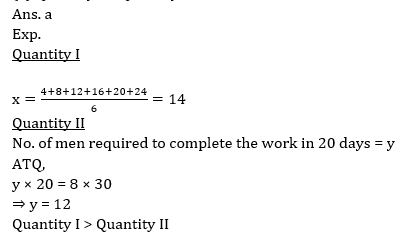6. Quantity I — cost price of an article having marked price = Rs. 400, which when sold at 20% discount still make a gain of 20/3%
Quantity II — cost price of an article which is sold at 14% profit and if cost price and selling price both are Rs. 117 less, the profit would be 9% more.
(a) if quantity I > quantity II
(b) if quantity I < quantity II
(c) if quantity I ≥ quantity II
(d) if quantity I ≤ quantity II
(e) if quantity I = quantity II or no relation can be established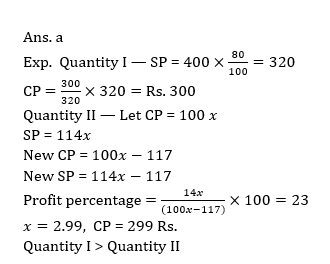7. Quantity I — the sum of money for which the difference between SI and CI obtained on it in 2 years at 6% per annum compounded annually is Rs. 43.2.
Quantity II — Rs. 12850
(a) if quantity I > quantity II
(b) if quantity I < quantity II
(c) if quantity I ≥ quantity II
(d) if quantity I ≤ quantity II
(e) if quantity I = quantity II or no relation can be established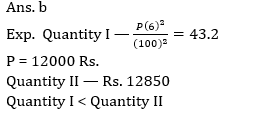8. Quantity I — average income of the whole group of 75 people, if average income of the men in the group is Rs. 4200 and that of women is Rs. 4000. (total men : total women = 8 : 7)
Quantity II — The average income of 20 people, which decreases by Rs. 150 if a person with income of Rs. 1000 joins them.
(a) if quantity I > quantity II
(b) if quantity I < quantity II
(c) if quantity I ≥ quantity II
(d) if quantity I ≤ quantity II
(e) if quantity I = quantity II or no relation can be established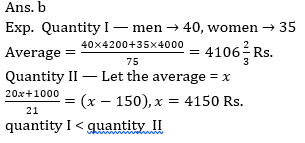9. Quantity I — The distance of school from Aman’s house if he reaches school 5 minutes late which walking at 4 km/hr but 10 minutes earlier than scheduled time walking at 5 km/hr.
Quantity II — 5 km
(a) if quantity I > quantity II
(b) if quantity I < quantity II
(c) if quantity I ≥ quantity II
(d) if quantity I ≤ quantity II
(e) if quantity I = quantity II or no relation can be established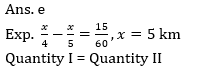10. Quantity I — Product of 2 numbers, whose sum is 17 and sum of the squares of 2 no. is 145.
Quantity II — Sum of 2 numbers, whose product is 1400 and difference between them is 5.
(a) if quantity I > quantity II
(b) if quantity I < quantity II
(c) if quantity I ≥ quantity II
(d) if quantity I ≤ quantity II
(e) if quantity I = quantity II or no relation can be established#### Attempt Quantitative Aptitude Topic Wise Online Test Series

Recommended PDF’s for:

#### Most important PDF’s for Bank, SSC, Railway and Other Government Exam : Download PDF Now

AATMA-NIRBHAR Series- Static GK/Awareness Practice Ebook PDF Get PDF here
The Banking Awareness 500 MCQs E-book| Bilingual (Hindi + English) Get PDF here
AATMA-NIRBHAR Series- Banking Awareness Practice Ebook PDF Get PDF here
Computer Awareness Capsule 2.O Get PDF here
AATMA-NIRBHAR Series Quantitative Aptitude Topic-Wise PDF Get PDF here
AATMA-NIRBHAR Series Reasoning Topic-Wise PDF Get PDF Here
Memory Based Puzzle E-book | 2016-19 Exams Covered Get PDF here
Caselet Data Interpretation 200 Questions Get PDF here
Puzzle & Seating Arrangement E-Book for BANK PO MAINS (Vol-1) Get PDF here
ARITHMETIC DATA INTERPRETATION 2.O E-book Get PDF here

3